## Physical units and constants

#### Physical constants (NIST, OEIS)

 Avogadro's constant NA 6.022 141 29(27) x 1023 mol-1 Boltzmann constant k, kB 1.380 6488(13) x 10-23 J K-1 Electric constant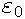8.854 187 817... x 10-12 N A-2 Elementary charge e 1.602176487(40) x 10-19 J m-1 Magnetic constant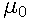4x 10-7 N A-2 Magnetic flux quantum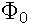2.067 833 758(46) x 10-15 Wb Molar gas constant R 8.314 4621(75) J mol-1 K-1 Pi3.14159265358979323846... Euler's number e 2.71828182845904523536... Planck's constant h 6.626 069 57(29) 10-34 Js Speed of light in vacuum c, c0 2.99792458 108 ms-1

## Physical Units

Energy
In physics, energy is a scalar quantity describing the amount of work that can be performed by a force. It is an attribute of objects and systems. Because of its definition, the SI unit for energy is the same as the unit of work - the joule (J), named after James Prescott Joule. 1 joule is equal to 1 newton-metre or in terms of SI base units: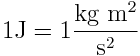Other units of energy include:
• The erg (measured in cgs units): 1 erg = 1 g cm2 / s2, which equals 1.0 x 10-7J.
• The electronvolt (sometimes electron volt) eV: this is a common unit for energy measurements on atomic scale, it is equal to the amount of energy gained by a single unbound electron when it accelerates through an electrostatic potential difference of one volt: 1 eV = 1.60217653(14) x 10-19J.
• In spectroscopy, people tend to use cm-1 since energy is inversely proportional to wavelength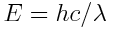.
• kWh: 1 kWh = 3.6 x 106J
• BOE/toe: the barrel/ton of oil equivalent, based on the approximate energy released by burning a barrel/tonne of crude oil. The BOE is defined as 6.1178632 x 109J, a toe is approximately 42 GJ.
Pressure
(symbol: p) is the force per unit area applied to an object in a direction perpendicular to the surface. The SI derived unit used for pressure, stress, Young's modulus and tensile strength is the pascal (Pa), named after Blaise Pascal. As a measure of force per unit area it is equivalent to to one newton per square meter or one joule per cubic metre:
1 Pa = 1 N / m2.
There are lots of different units used for pressure measurement:
The standard atmosphere (atm) is an international reference pressure defined as 101325 Pa (just to confuse me further, there is one more unit called the technical atmosphere (at), defined as 98.0665 kPa, ≈ 0.96784 standard atmospheres).
The torr, named after Evangelista Torricelli is defined as 1/760 of an atmosphere.
The bar is a non-SI unit, but accepted for use with the SI. It is defined as 1 bar = 100 kPa (kilopascals) = 105 Pa.
The psi (pound-force per square inch, also lbf/in2 or lbf /in2) is a unit of pressure or of stress based on avoirdupois units. 1 Pa is ≈ 1.4504 x 10-4 psi .

## Some handy conversions

• Convert wavelength (measured in the SI unit m) to energy (in SI unit J or in eV):

Here's the story: our wave has an energy of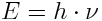. Furthermore, frequency and wavelength are linked via the speed of light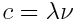. This leads to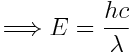where the values used are
c = speed of light in vacuum = 2.99792458 x 1010 cm/sec = 2.99792458 x 108 m/sec
h = Planck's constant = 6.626 x 10-34 J/sec
A wave in vacuum with a wavelength of
m has a frequency of   5.451e+14 1/s (or Hz) and an energy E = 3.612e-19 J = 2.254e+0 eV
• Convert pressure units (SI unit of pressure is Pa):
There is a whole zoo of pressure units for historical reasons. Here is a list (probably incomplete) to convert from one to another
Pa     = 1.0e-5 bar     = 9.8692e-6 atm     = 7.5006e-3 Torr.
bar     = 1.0e+5 Pa     = 9.8692e-1 atm     = 7.5006e+2 Torr.
Torr     = 1.3332e+2 Pa     = 1.3332e-3 bar     = 1.3158e-3 atm.

## Links on constants and units

Notes:

1 In a lecture from 1961, Richard Feynman apologized to his students for using electron volts to measure energy instead of some multiple of the joule (see wikipedia).

2 The pound (lb) used is the international avoirdupois pound, defined to be exactly 0.45359237 kg. The pound-force (lbf) approximately equals the force exerted on a mass of one lb on the surface of earth. Using standard gravity g = 9.80665m/s2, one can calculate the pound-force:

1 lbf = 0.45359237 kg 9.806650 m/s2 = 4.448221615 kg m/s2
One inch (in) is exactly 2.54 cm, so a pascal can be written as
1 Pa = (0.0254 m/in)2 / 4.448221615 N/lbf = 1.4504 x 10-4 psi